Recall the "normal" probability distribution: $$\qquad\qquad{\rm gaussian}(x)=\frac{1}{\sigma\sqrt{2\pi}}{\rm e}^{\textstyle-\frac{(x-\mu)^2}{2\sigma^2}}$$

In :
def gaussian(x,mu=0.,sigma=1.):
return exp(-(x-mu)**2/(2.*sigma**2))/(sigma*sqrt(2.*pi))

In :
x=arange(-5,5.1,.1)
y=gaussian(x)
plot(x,y)
xticks(range(-4,5))
xlim(-4,4);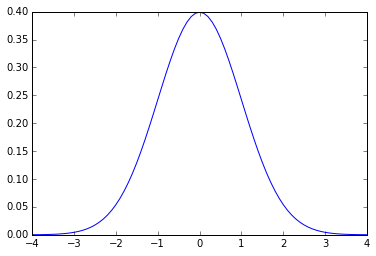To get a rough feeling for the fraction of the area under the curve, we can approximate the integral by doing a sum, with stepsize of .0001 (note that since the function is symmetric, we can integrate from 0 to xmax and double the result, rather than sum all the way from -xmax to xmax):

In :
def q(xmax): return 2*.0001*sum(gaussian(arange(0,xmax,.0001)))


Since it's a probability distribution, the total integral under the curve is 1, and the fractional values for +/- 1,2,3 standard deviations $\sigma$ from the mean reproduce the "68–95–99.7" rule:

In :
[(sigma,round(q(sigma),5)) for sigma in range(1,5)]

Out:
[(1, 0.68271), (2, 0.95453), (3, 0.99734), (4, 0.99998)]

These are related to "z-values" which can be found tabulated in standard tables. (Note that the value for $\sigma=2$ rounds to .95 — the more accurate value for an exact .95 confidence interval is closer to $\sigma=1.96$.) The above values are twice the cumulative from mean (0 to Z), since they include both above and below the mean.

In :
figure(figsize=(8,4))
x=arange(-5,5.1,.1)
y=gaussian(x)
plot(x,y)
fill_between(x,y,color='blue',where=abs(x)<3.01)
fill_between(x,y,color='#00AAAA',where=abs(x)<2.01)
fill_between(x,y,color='cyan',where=abs(x)<1.01)
for i,iy,pct in (1,.235,' 68'),(2,.05,' 95'),(3,.02,'99.7'):
annotate(pct+'%',xytext=(i+(.4 if i < 3 else .46),iy),xy=(.4,iy),
arrowprops={'arrowstyle':"<-"},ha='center',va='center',fontsize=14)
annotate('$\pm'+str(i)+'\sigma$',xy=(-(i+.05),iy),xytext=(0,iy),
arrowprops={'arrowstyle':"->"},ha='center',va='center',fontsize=15)
xticks(range(-4,5))
xlim(-4,4);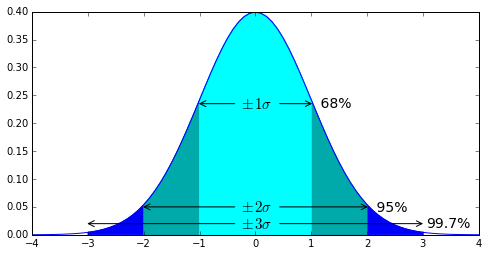We can also define a function p(xmax) as the area under the curve for x > xmax (though note that p(xmax) could also be defined in terms of q(xmax) since by symmetry q(xmax)+2p(xmax)=1 ):

In :
def p(xmax): return .0001*sum(gaussian(arange(xmax,10,.0001)))


We see that values of x more than roughly 1.645 standard deviations above the mean correspond to the typically used p-value of .05:

In :
round(p(1.645),4)

Out:
0.05

This values can also be obtained from "z-values" tabulated in standard tables. The usual cumulative z-value gives the probability that a statistic is less than z, and the probability of being above that value is called the complementary cumulative, equal to 1 minus the cumulative z-value. (So the cumulative z-value of 1.645 is roughly .95, and the complementary cumulative z-value is .05 .)

In :
x=arange(-5,5.1,.05)
y=gaussian(x)
plot(x,y)
fill_between(x,y,color='r',where=x>1.64)
annotate('$1.645\sigma$',xytext=(.5,.08),xy=(1.645,.08),
arrowprops=dict(arrowstyle="->"),ha='center',va='center',fontsize=15)
annotate('5%',xytext=(2.5,.08),xy=(2,.02),
arrowprops=dict(arrowstyle="->"),ha='center',va='center',fontsize=14)
xticks(arange(-4,5));
xlim(-4,4);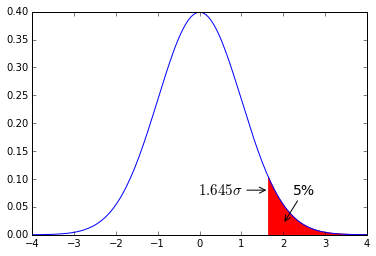Finally, a quick look at the Central limit theorem: consider a set of $N$ independent random variables $X_i$, with arbitrary probability distributions $p_i(x)$, with means $\mu_i$ and variances $\sigma_i^2$, If we consider the sum $X=\sum_{i=1}^N X_i$, then for sufficiently large $N$, and whatever the other properties of those independent probability distributions, the probability of $X$ will tend to a gaussian (normal) distribution with $\mu=\sum_{i=1}^N \mu_i$ and variance $\sigma^2=\sum_{i=1}^N\sigma_i^2$.

As an illustration, we'll consider a set of $N=100$ biased coins, given by the following array of probabilities for flipping heads:

In :
N=100
ps=rand(N)
print ps
m=sum(ps)
s=sqrt(sum(ps*(1-ps)))
print '\n sum = ',m,', sqrt of sum of variances=',s

[  5.69170818e-01   8.67317321e-01   6.66190386e-01   2.98615928e-01
2.16640163e-01   9.07432348e-01   4.65905118e-01   9.39634488e-01
8.18324716e-02   1.64673829e-01   5.10504864e-01   4.75663720e-01
5.86620705e-01   3.64455305e-01   1.45011289e-02   6.65831850e-01
4.47445059e-01   3.08840076e-01   3.30986516e-01   6.80786463e-01
4.10972309e-01   4.10871930e-01   7.42533642e-01   4.42544269e-02
1.36767530e-01   8.57689528e-01   9.48110526e-01   4.93218187e-01
4.32564845e-02   6.84323916e-01   3.93547920e-01   4.67854357e-01
1.06940518e-01   9.62199663e-01   3.08810573e-01   8.93916702e-01
7.62989511e-01   3.47333936e-01   3.91478825e-01   8.75229484e-01
4.19158208e-02   2.44692077e-01   7.56443810e-01   7.41190405e-02
4.58250678e-01   6.69825429e-01   7.80104641e-01   8.09701763e-01
8.95061322e-02   6.12538795e-01   6.70219482e-01   1.30603654e-02
5.00404869e-01   2.74923459e-01   1.68611343e-01   1.22729215e-01
6.90070397e-01   8.75336794e-01   6.42762121e-01   4.99318009e-01
2.26807512e-01   6.74476531e-01   5.42228998e-01   2.57091495e-01
1.34922835e-01   2.03299768e-01   9.91107966e-01   4.41279185e-01
2.15137178e-01   6.75745280e-01   6.29044299e-01   7.57308471e-01
1.13220670e-01   9.10278417e-01   2.81916025e-01   2.34356596e-01
6.60694147e-02   3.01243888e-01   1.94465041e-01   6.69993652e-01
1.17672630e-01   1.72354766e-01   3.61265695e-01   8.58106032e-01
2.59886545e-04   6.63260747e-01   8.28243635e-01   2.15207409e-01
5.05777674e-01   3.51390766e-01   2.79628882e-01   8.66800091e-01
4.26554671e-01   7.84459506e-01   5.67139702e-02   6.55103683e-01
1.91337638e-01   5.94856297e-01   6.77305971e-01   6.19628490e-01]

sum =  46.5858044316 , sqrt of sum of variances= 4.1297714602


(Note that rand() is a "convenience function", for random.random(), and generates an array of random numbers valued between 0 and 1.)

We flip each coin by picking a random number between 0 and 1, and if that number is less than the "bias" of the coin, then we record a flip of heads for the variable $X_i$. The variable $X$ is then the sum of the number of recorded heads for the 100 flips. We do 100000 trials of 100 flips apiece:

In :
trials = 100000
def rtrial(): return sum(rand(N) < ps)
results = [rtrial() for t in xrange(trials)]
print mean(results),std(results)

46.57011 4.11410556353


We see these compare well to the above values expected from the central limit theorem, and the probability distribution for $X$ is gaussian, even though it is composed from the sum of one hundred different (and non-gaussian) random variables:

In :
figure(figsize=(8,4))
hist(results,arange(-.5,101),label='data');
xg=arange(int(m-4*s)+1,int(m+4*s),.1)
yg = gaussian(xg,m,s)
plot(xg,trials*yg,'r-',label='gaussian',linewidth=1.5)
plot((m,m),(0,trials*gaussian(m,m,s)),'y--')
annotate('',xytext=(m-s,trials*gaussian(m-s,m,s)),xy=(m+s,trials*gaussian(m+s,m,s)),
arrowprops=dict(arrowstyle="<->"))
title('data fit by gaussian with predicted mean={}, stdev={}'.format(round(m,1),round(s,1)))
xlim(int(m-4*s)+1,int(m+4*s));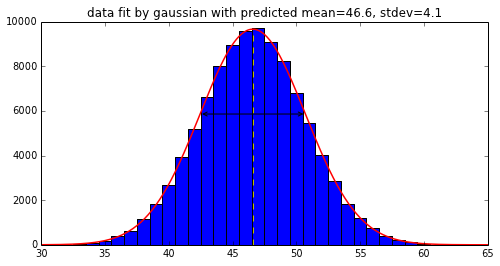Note that N needn't even be as large as 100 for the central limit theorem to work reasonably well -- retry the above, e.g., for N=30.

For another example of arbitrary probability distributions combining to form a normal distribution, consider a population with bimodal heights: half this population has height of exactly 5.5 feet, and the other half has height of exactly 6 feet. We choose N people at random and measure the average of their heights. That average will be normally distributed, with a mean of 5.75 and a standard deviation of sqrt(N/16.)/N $=1/4\sqrt N$.
(1/16. is the variance of the above distribution, since each of the possibilities is 1/4. from the mean, and the additional factor of N in the denominator is because we are considering the mean rather than the sum.) For N=100, the std is 1/40. = .025 .

In :
def height(n):
return [6 if tall else 5.5 for tall in random.random(n) > .5]

In :
N=100
trials=100000
results = [mean(height(N)) for t in xrange(trials)]

In :
print mean(results),'expected mean',5.75
print std(results),'expected std',1/40.

5.7500644 expected mean 5.75
0.0250118542423 expected std 0.025


We see that the mean and std of the distribution are as expected, and in addition the full probability distribution is well described by a normal distribution (where the average heights over 100 people can take fractional values in between 5.5 and 6 even though any individual height is one of those two extremes with equal probability):

In :
step=.005
hist(results,arange(5.5-step/2,6.1,step),label='data');
xg=arange(5.6,6,step/5)
yg=step*trials*gaussian(xg,5.75,1./40)
plot(xg,yg,'r')
xlabel('average height of '+str(N)+' bimodal people')
xlim(5.65,5.85);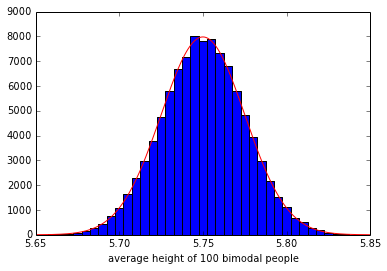As a last example, consider measuring samples of the population for some trait. Standard red/green color blindness affects roughly 8% of males (the relevant gene that codes for the pigment in the retinal cone cells is on the X chromosome, so is sex-linked, and only .6% of females are affected since that would require two of the variant X chromosomes, .08*.08=.0064). Suppose that 194 boys in an incoming class of high school students are tested for color blindness, what range of results is expected?

We're considering drawing the sample at random from some large population, so the result of the test can be considered a random variable with a probability of .08 of testing positive. For a set of n samples with probability p, define:

In :
def rn(p,n): return sum(random.random(n) <= p)


This will return the total number of n that test positive, if each has a probability of p of testing positive.

These results should be roughly normally distributed, so if we do some large number of trials:

In :
trials=100000
results = [rn(.08,194) for t in xrange(trials)]

In :
print mean(results),'expected',.08*194
print std(results),'expected',sqrt(.08*.92*194)

15.53083 expected 15.52
3.77356191298 expected 3.77867701716


We see that the distribution is roughly a normal distribution:

In :
hist(results,bins=arange(-.5,36))
xg=arange(0.,35.,.1)
yg=trials*gaussian(xg,.08*194,sqrt(.08*.92*194))
plot(xg,yg,'r-')
for b in 3.5,7.5,23.5,27.5: plot((b,b),(0,trials/20),'k--')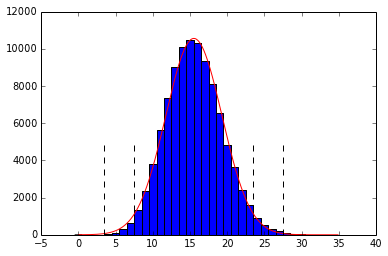where the expected mean $\pm 2$ and $\pm 3$ standard deviations are indicated by dotted lines.
From the '68-95-99.7' rule, it's very likely (95%) that the results of a single measurement will fall between $15.52 \pm 2\cdot 3.78$, so from 8 to 23, and almost certain (99.7%) that they'll fall between $15.52 \pm 3\cdot 3.78$, so from 4 to 27 measured to have color blindness.

Additional note: looking carefully at the above graph, systematic deviations from a normal distribution are evident (the actual data is shifted slightly to the left of the red line near the maximum). This is in part because the mean of 15.5 is small enough that it's better described by a Poisson distribution. On the other hand, if we're only trying to estimate the likely range of results as within three standard deviations from the mean, then from the graph using a normal distribution is clearly good enough.

To compare instead with a Poisson distribution, the following code could be added:

from scipy.misc import factorial
def poisson(z,m): return exp(-z)*z**m/factorial(m)
yg=trials*poisson(.08*194,xg)
plot(xg,yg,'y-')

It is also possible to draw from a normal distribution, the function random.normalvariate() generates random pulls from a specified mean and standard deviation, as does numpy.random.normal() (and as well scipy.stats.norm.rvs(), see below):

In :
from random import normalvariate
normalvariate(0,1),normalvariate(100,20)

Out:
(1.2483312609153183, 91.68889999099383)
In :
from numpy.random import normal
normal(0,1), normal(100,20)

Out:
(-0.3617152617544284, 139.38243360023566)

Many such pulls these can be generated either via loop

    [normalvariate(0,1) for i in xrange(10)]


(or with an equivalent function norm() from scipy.stats):

In :
trials=1000
xdata=np.arange(-4,4,.1)
plt.plot(xdata,trials*.1*gaussian(xdata),'r')
plt.hist([normalvariate(0,1) for t in xrange(trials)],bins=xdata);
#same thing:
#hist(norm.rvs(0,1,trials),bins=xdata)
None;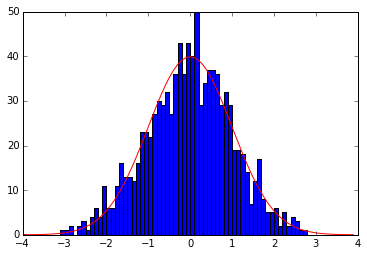In :
from scipy.stats import norm
#http://docs.scipy.org/doc/scipy-0.14.0/reference/generated/scipy.stats.norm.html
#has some other useful methods, e.g.
#rvs random variates
#pdf prob density function: same as the exp(-...) in gaussian() above
#cdf cumulative density function: the integral under curve from -infinity to x (q(x)/2 + 1/2)
#ppf percent point function -- inverse of cdf, to get x for given pct

In :
x = np.linspace(-3, 3, 101)
plt.plot(x,norm.pdf(x));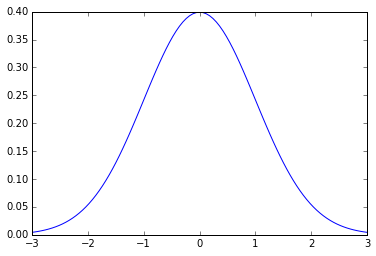In :
norm.rvs(0,1,10)

Out:
array([-0.26953132,  1.42484678, -0.19684232, -0.32139534,  0.43598938,
0.03922205,  0.09901545,  0.94656892, -0.3505457 ,  0.81771766])
In :
print norm.ppf(0.05),norm.ppf(0.95) #get std dev for p=.05, 90% within
print norm.ppf(.1), norm.ppf(.9) # 80% within boundaries

-1.64485362695 1.64485362695
-1.28155156554 1.28155156554

In :
#same function as q above, defined for you
def prob_between_pm(x): return 2*norm.cdf(x)-1
for i in range(1,9): print i,prob_between_pm(i)

1 0.682689492137
2 0.954499736104
3 0.997300203937
4 0.999936657516
5 0.999999426697
6 0.999999998027
7 0.999999999997
8 1.0

In :
def pmx_for_prob(x): return norm.ppf(x/2. + .5)
pmx_for_prob(.8), pmx_for_prob(.9)  #for 80% and 90% confidence intervals

Out:
(1.2815515655446004, 1.6448536269514722)
In :
plt.plot(x,norm.cdf(x))
plt.plot(x[x>=0],prob_between_pm(x[x>=0]))
plt.grid('on');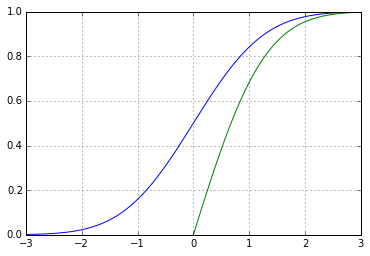At the end of class, we considered the example of a sample poll of n=1000 people, of whom k=550 answered 'Yes' to some question.

First recall that we can consider either the standard deviation of the number count, or the standard deviation of the percentage.

The standard deviation of the number count for a Bernoulli trial consisting of n events each with probability $q$ of success was derived in class to be $\sigma=\sqrt{nq(1-q)}$. That means that if the actual probability is $q$, then we would expect to get $nq$ Yes responses, and if we keep redoing the poll with different samples of n people taken from the same distribution, then 68% of the time we would expect to get values of k between $nq-\sigma$ and $nq+\sigma$. If we do the poll only once and get k yes votes then our estimate of the underlying probability is p=k/n, but it could by off due to the finite sample size. If we keep redoing the poll many times and average the values of p, they will get closer and closer to the underlying probability $q$.

Since p=k/n, the standard deviation of the estimated probability is given by dividing the standard deviation of the number count by n: $\sqrt{np(1-p)}\ /\ n = \sqrt{p(1-p)/n}$. Notice that the n is now in the denominator under the square root. (That is because the standard deviation of the number count had a factor of n in the numerator under the square root, and when we divide by n that gets converted into a factor of n in the denominator under the squareroot: $\sqrt{n}\ /\ n = \sqrt{1/n}$.)

Here is a slightly longer explanation of how the error estimate for the number count can be turned around into an error estimate for the underlying probability distribution. Assuming the 1000 people are drawn at random from some very large population (for some details of the assumptions that go into this, see sampling), what is the likely percentage of 'Yes' voters in the full population? The idea is that the fraction p=550/1000=.55 is sampled from some much larger population whose overall fraction is some unknown value q. Each sampled person can be considered to be a Bernoulli process with probability q of success, and therefore the number of 'Yes' voters is normally distributed with mean q and standard deviation sqrt(n*q(1-q)). Say the range of q of interest is that from which we could have drawn with at least 90% probability the 550 'Yes' votes of 1000 and inferred p=.55 . We know that values of k within 1.645 standard deviations from the mean n*q will occur 90% of the time, so the range of interest is values of q such that p=k/n is within 1.645 standard deviations above or below. We don't know the real q so can't calculate its $1.645\sigma$ precisely, but q will be close enough to p that we can estimate $\sigma=$ sqrt(p(1-p)/n), and $1.645\sigma=1.645*\sqrt{.45*.55/1000}\approx.026$. (Note that the n has switched to the denominator inside the square root, because we are considering the standard deviation of k/n, hence divide the standard deviation of k by n.) The range of q that could give the inferred value of p=.55 with 90% likelihood is thus given by q between the values $.55\pm.026$, as in the simulation below (where the mean of the normal distribution is animated to range between those two values, and the observed p is fixed at .55):

In :
from urllib2 import urlopen
from IPython.display import Image

Out: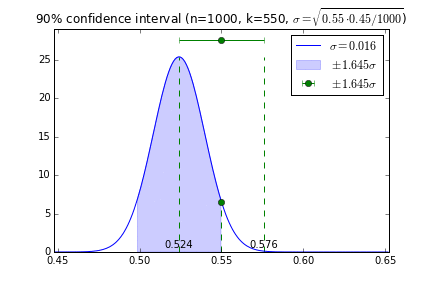For values of q either below .524 or above .576, the total probability of finding 550 'Yes' voters falls below 10%, because it is too large a fluctuation from the mean in a sample of 1000. (A hidden assumption in this has been that all values of q are a priori equally likely, which implied as well that the mean of the true normal distribution is equally likely to be above or below the observed sample mean.)

There is an on-line "margin of error" calculator, which calculates the 95% confidence interval (corresponding to $1.96\sigma$) for an evenly split sample (probability p=.5). For a sample of n=1000 and a large overall population size, say N=1000000, the "margin of error" calculator should give 1.96* sqrt(.5*.5/1000) = .031 (try it), and as long as the overall population size N is at least roughly 20 times larger than the sample size n, the calculator should continue to give a margin of error of 1.96/sqrt(2*2*n)=.98/sqrt(n), independent of the overall size of the population. As the sample size becomes comparable to the overall population size, however, the margin of error decreases, until the limiting value of sample size equal to population size, at which point the margin of error is zero (the entire population is sampled). The exact value for the standard deviation of drawing n samples (with no repeats) from a population of N is given by $\sqrt{{1-n/N\over 1-1/N}q(1-q)}$, where the first factor is known as the "finite population correction" (and can be derived by considering the probability distribution for the hypergeometric distribution, the simple modification necessary for "sampling without replacement", i.e., not asking the same person more than once, which becomes more likely as the sample size becomes an appreciable fraction of the total population). Note that the extra factor is zero when n=N, and is irrelevant when N is large and much greater than n.
You can also check to see whether the above calculator has implemented this correction factor properly by considering the intermediate range of values of n/N.

For reference, this is the code used to produce the plots for the above animated .gif:

In :
p=.55
n=1000
j=0  #png counter
sigma=sqrt(p*(1-p)/n)
ci90=1.645*sigma  #90% confidence interval
h=gaussian(0,0,sigma) #value of gaussian at peak
x=arange(p-6.5*sigma,p+6.5*sigma,.001)
for q in arange(p-ci90,p+ci90+.001,2*ci90/23):
figure(figsize=(6,4))
errorbar(p, 27.5, xerr=ci90, fmt='go', label='$\pm1.645\sigma$')
y=gaussian(x,q,sigma) #centered at q, std=sigma
plot(x,y,'b',label='$\sigma={:.3f}$'.format(sigma))
fill_between(x,y,color='b',alpha=.2,where=abs(x-q)<1.65*sigma)
ch=gaussian(p,q,sigma) #value of q-centered gaussian at p=.55
vlines((p-ci90,p,p+ci90),0,(h,ch,h),color='g',linestyles='--')
plot(p,ch,'go')
for pl in (.524, .576): text(pl,.5,pl,ha='center')
xlim(p-6.5*sigma,p+6.5*sigma),ylim(0,29)
title('90% confidence interval (n={0:}, k={1:}, $\sigma=\sqrt{{{2:}\cdot{3:}/{0:}}}$)'.format(n,int(p*n),p,1-p))
# label='' on the fill_between is broken, so a non-visible hack for the legend:
fill((0,.1,0),(5.1,5.0,4.9), color='b', alpha=.2,label='$\pm1.645\sigma$')
legend(numpoints=1)
savefig('pngs/img{:03d}.png'.format(j))
j+=1
close();
## then convert -delay 20 -loop 0 img*.png ci90.gif

In [ ]: